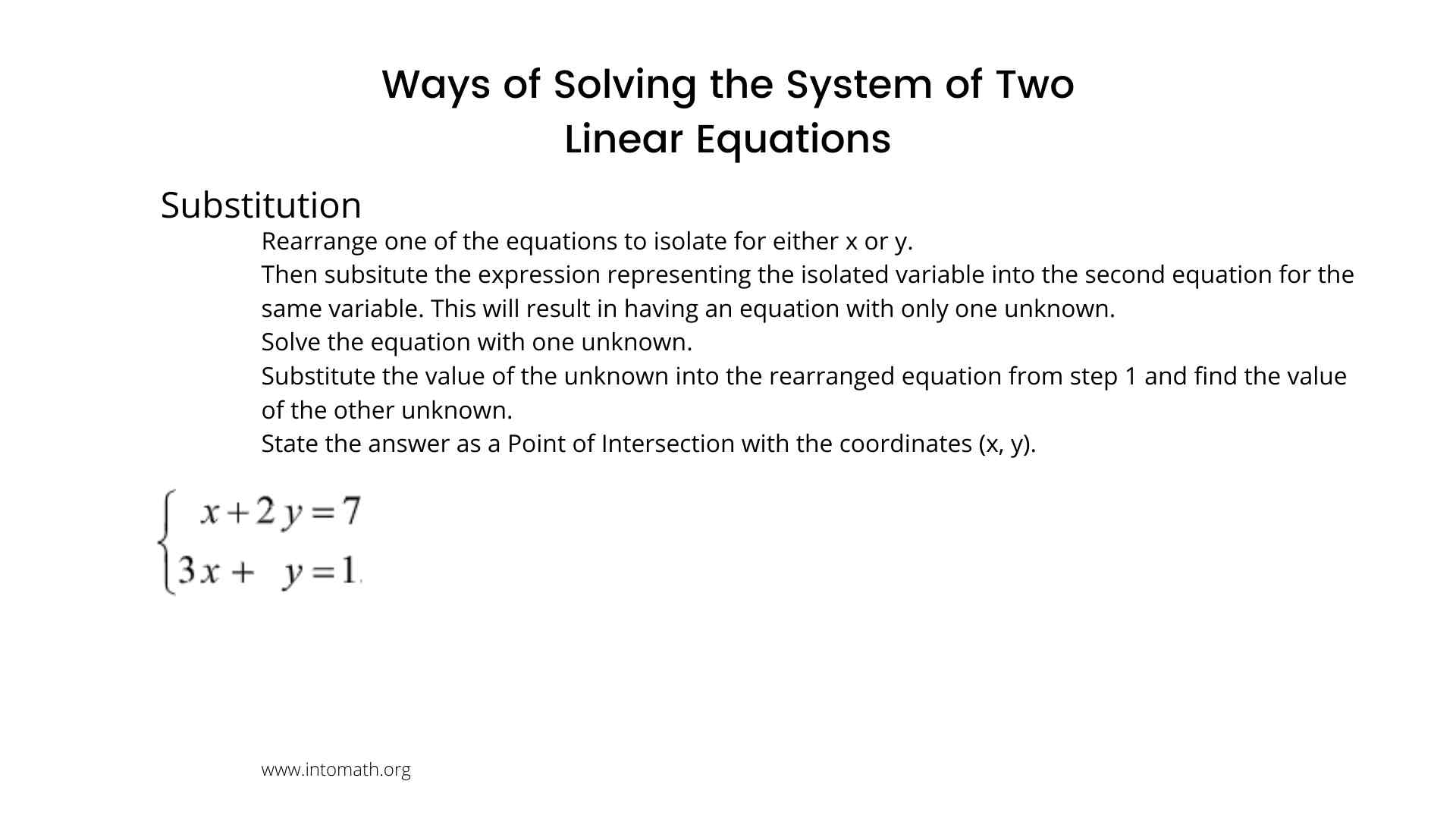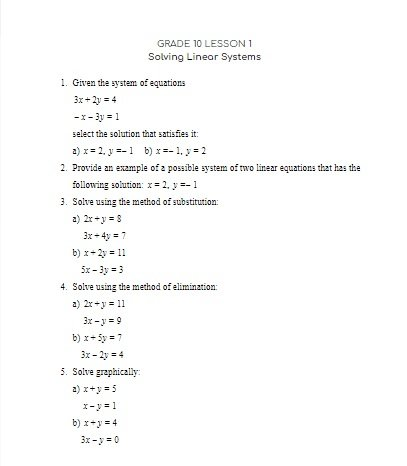CA\$2.00

A system of linear equations is when two or more equations are considered at the same time and we are looking for the point of intersection of the equations. In Grade 10 we are only looking at the system of 2 equations.

We are learning how to solve a system of linear equations in three ways: graphically, by substitution and by elimination.

To solve the system of linear equations with two unknowns means to determine the values of the unknowns, that satisfy BOTH linear equations in the system or show that the solution does not exist.

The System Solution = Point of Intersection of the two lines.

If two lines are parallel, the system will have no solutions.

When we want to solve the system graphically, we graph the equations of the lines and determine the point of intersection visually on the graph. this may not be accurate.

Elimination is a method when equations are being added or subtracted. One of the variables gets eliminated. Then the new single equation is solved for one of the variables. After that, the result is used to determine the value of the other variable.